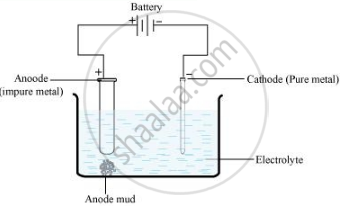PUC Karnataka Science Class 12Department of Pre-University Education, Karnataka
Share

Books Shortlist

Explain the principle of the method of electrolytic refining of metals. Give one example. - PUC Karnataka Science Class 12 - Chemistry

Questions

Explain the principle of the method of electrolytic refining of metals. Give one example.

Outline the principles of refining of metals by the following methods: Electrolytic refining

Write the principle of electrolytic refining.

Solution 1

Electrolytic refining:

Principle: In this method, an anode of a block of impure metal and a cathode of a thin sheet of pure metal, are suspended in an electrolyte solution which is the solution of a soluble salt of the metal usually a double salt of the metal. For example, the purest copper is obtained by an electrolytic refining method. A slab of impure copper as the anode and a thin sheet of pure copper as the cathode are dipped in the electrolyte which is an acidic solution of copper sulphate. On passing electricity through the cell, copper is dissolved from the anode and deposited on the cathode.

Anode: Cu(s) → Cu 2+ (aq) + 2e-

Cathode: Cu2+ (aq) +2e- → Cu (s)

The impurities of iron, nickel, zinc and cobalt present in blister copper being more electropositive pass into solution as soluble sulphates. The impurities of antimony, selenium, tellurium, silver, gold and platinum being less electropositive are not affected by CuSO4-H2SO4 solution. Therefore, these impurities get settle down under the anode as anode mud or anode sludge.

Solution 2

Electrolytic refining - Electrolytic refining is the process of refining impure metals by using electricity. In this process, impure metal is made the anode and a strip of pure metal is made the cathode. A solution of a soluble salt of the same metal is taken as the electrolyte. When an electric current is passed, metal ions from the electrolyte are deposited at the cathode as pure metal and the impure metal from the anode dissolves into the electrolyte in the form of ions. The impurities present in the impure metal gets collected below the anode. This is known as anode mud.

Anode :  M -> M^(n+) + "ne"^-

Cathode: M^(n+) + "ne"^(-) -> MSolution 3

Electrolytic refining:
Electrolytic refining is the process of refining impure metals by using electricity. In this process, impure metal is made the anode and a strip of pure metal is made the cathode. A solution of a soluble salt of the same metal is taken as the electrolyte. When an electric current is passed, metal ions from the electrolyte are deposited at the cathode as pure metal and the impure metal from the anode dissolves into the electrolyte in the form of ions. The impurities present in the impure metal gets collected below the anode. This is known as anode mud.

Anode :  M -> M^(n+) + "ne"^-

Cathode : M^(n+) + "ne"^(-) -> M

Is there an error in this question or solution?

APPEARS IN

NCERT Solution for Chemistry Textbook for Class 12 (2015 to Current)
Chapter 6: General Principles and Processes of Isolation of Elements
Q: 26 | Page no. 164
Solution Explain the principle of the method of electrolytic refining of metals. Give one example. Concept: Refining.
S